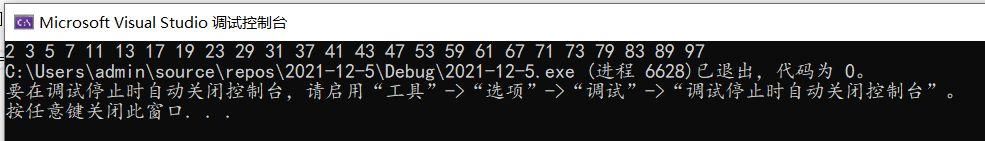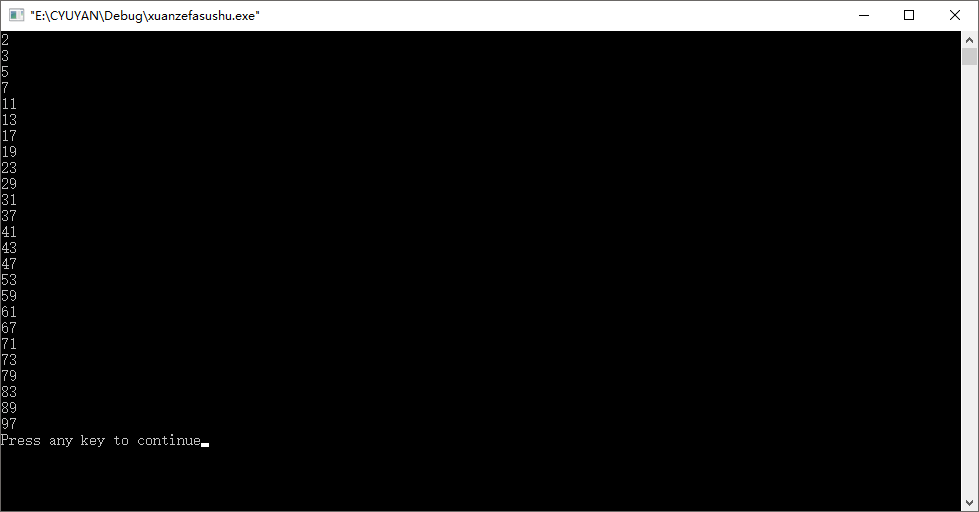• 用筛选法求100以内素数
2021-05-22 08:56:49

使用筛选法求100以内的素数

筛选法介绍

先把N个自然数按次序排列起来。 1不是质数，也不是合数，划去。 第二个数2是质数留下来，而把2后面所有能被2整除的数都划去。2后面第一个没

划去的数是3，把3留下，再把3后面所有能被3整除的数都划去。3后面第一个没划去的数是5，把5留下，再把5后面所有能被5整除的数都划去。这样一直做下去，就会把不超过N的全部合数都筛掉，留下的就是不超过N的全部质数。

这其实是一种很粗暴的解法, 去逐个整除质数!

程序流程

* 将N个自然数写入到一个数组中, 1划掉(设置为0)

* 2后面所有能够整除2的数, 划掉(置0)

* 设2后面的第一个非0数就是第二个质数设置为N, 将N后所有能够整除N的数, 划掉

* 以此类推

实际代码

#include

#include

#include

static void prime_number(int num)

{

std::vector pm(num);

for(int i = 0; i < num; i++)

{

pm[i] = i + 1;

}

pm = 0; //1不是素数

for(int i = 1; i < num - 1; i++)

{

for(int j = i + 1; j < num; j++)

{

if(pm[i] && pm[j] && 0 == pm[j] % pm[i])

{

pm[j] = 0;

}

}

}

int k = 1;

for(auto c : pm)

{

if(c)

{

printf("%d\t", c);

k++;

}

if(k % 10 == 0)

{

printf("\n");

k++;

}

}

printf("\n");

}

int main()

{

prime_number(128);

system("pause");

return 0;

}

上一次写字符串RK算法时, 计算hash可以以每一个字符指代要给素数更好, 当时查了一下素数的求解方式

不过感觉实际没有必要去求一定数量的素数, 因为如果少量的话直接求出来放在数组里面就可以了, 非常多的话那么算法也绝不是这么简单!

更多相关内容
• 此时，3后面的数变成了5，再剩下的数去除5……以此类推，直到剩下的全为素数。 而如何“去除”不是素数的数呢？我们可以将那些数找出来，统统赋上一个值，最后打印出这个数组中不是这个值的数就好了 int main() ...•  首先，1不是素数。
• 从2开始，将后面的每个数都除以2，如果能整除说明其一定不是素数，将这些数去除。此时2后面紧跟着的就是3，再将剩余的这些数除以3，将可以整除的数全部去除；此时，3后面的数变成了5，再用剩下的数去除5……以此类推，直到剩下的全为素数。
• 而如何“去除”不是素数的数呢？我们可以将那些数找出来，统统赋上一个值，最后打印出这个数组中不是这个值的数就好了
int main()
{
int arr = {0};
int i = 0;
int j = 0;
for (int i = 0; i < 100; i++)  //对数组进行初始化
{
arr[i] = i + 1;
}
arr = 0;  //因为1绝对不是素数，故可直接令其=0
for (i = 1; i < 100; i++)
{
if (arr[i] != 0)
{
for (j = i + 1; j < 100; j++)
{
if (arr[i] != 0 && arr[j] != 0)  //二者均不为0才进行取余操作，否则容易造成溢出
{
if (arr[j] % arr[i] == 0)    //将不是素数的数字定为0
arr[j] = 0;
}
}
}
}
for (i = 0; i < 100; i++)
{
if (arr[i] != 0)
printf("%d ", arr[i]);
}
return 0;

展开全文c++ c语言 c#
• //C语言 筛选法求100以内素数 //原理很简单，就是当i是质(素)数的时候，i的所有的倍数必然是合数。如果i已经被判断不是质数了，那么再找到i后面的质数来把这个质 //数的倍数筛掉。 //我们将从2开始 2除后面的数...

算法思路:

原理很简单，就是当i是质(素)数的时候，i的所有的倍数必然是合数。如果i已经被判断不是质数了，那么再找到i后面的质数来把这个质数的倍数筛掉。

代码如下:

//C语言 筛选法求100以内的素数
//原理很简单，就是当i是质(素)数的时候，i的所有的倍数必然是合数。如果i已经被判断不是质数了，那么再找到i后面的质数来把这个质
//数的倍数筛掉。
//我们将从2开始 用2除后面的数，如果后面的数能被2整除 那么那个数一定不是素数
#define N 100
#include "stdio.h"
int main(){
int i,j;
int arr[N];

for(i = 0;i<N;i++){//对数组赋值  1-100
arr[i]=i+1;
}
arr = 0;//1不是素数，所以将下标0 的元素设置为0

//进行素数判断:我们将从2开始 用2除后面的数，如果后面的数能被2整除 那么那个数一定不是素数,将那个数置为零
for(i = 1;i < N-1;i++){
for(j = i+1;j < N;j++){
if(arr[i] != 0 && arr[j] != 0)//如果进行到3的时候2后面一定有数被置为0了，这里我们需要判断一下是不是有0
if(arr[j] % arr[i] == 0){
arr[j] = 0;
}
}
}
//循环输出
for(i = 0;i<N;i++){
if(arr[i] != 0)
printf("%d\n",arr[i]);

}

return 0;
}

结果如下:展开全文• //筛选法就是“埃拉托色尼筛法” //就是将非素数“挖掉”，只剩下素数，所谓挖掉就是把非素数归为0，之后 进行判断即可 //1：先输入数组元素 //2：先把a等于0； //3：i从2开始，可以小于100，也可以根号下(100)...
//筛选法就是“埃拉托色尼筛法”
//就是将非素数“挖掉”，只剩下素数，所谓挖掉就是把非素数归为0，之后	进行判断即可
//1：先输入数组元素
//2：先把a等于0；
//3：i从2开始，可以小于100，也可以用根号下(100)来做，过程一样
//4：找2的倍数，3的倍数，四的倍数。。。。。
//如果可以整除则为0
//具体看代码

#include <stdio.h>
#include <math.h>
int main(void)
{
int a;
int i,j;
for(i=1;i<=100;i++)
{
a[i]=i;
}
a=0;//1不是素数
for(i=2;i<sqrt(100);i++)//进行sqrt(100)次比较
{
for(j=i+1;j<=100;j++)//假如i=2，则j从3开始到100，找出2的倍数，之后挖掉，以此类推
{
if(a[i]!=0&&a[j]!=0)
{
if(a[j]%a[i]==0)
{
a[j]=0;
}
}
}
}
for(i=2;i<100;i++)
{
if(a[i]!=0)
{
printf("%d\t",a[i]);
}
}
return 0;

}展开全文算法 c语言
• 使用筛选法来确定100以内素数并将其输出 使用时请在dev运行
• MIPS汇编下用筛选法求100以内素数，有C语言对照，工具的话，spim模拟器。MIPS
• 用筛选法求100以内的的素数 1.算法介绍 筛选法是指埃拉托色尼（Eratosthenes）筛选，埃拉托色尼是古希腊的著名数学家。他采取的方法是，先写下1到1000的全部整数，然后逐个判断它们是否素数，找出一个非素数，就把...c语言 算法
• int main() { int i,j,n,a;//为了使数和下标一一对应 ...=100;i++) { a[i]=i; a=0; } for(i=2;i<sqrt(1.0*100);i++) { for(j=i+1;j<=100;j++) { if(a[i]!=0 && a[j]!=0)...
• 所谓筛选法，指的是"埃拉托色尼筛法",采取的方法是，在一张纸上写上1-100 全部的整数 然后逐个判断他们是否为素数，找出一个非素数，就把他挖掉，最后剩下的就是素数 具体做法如下所示： 先把1挖掉，因为1不是素数 ...
• 什么是筛选法 所谓筛选法是指“埃拉托色尼筛法”，埃拉托色尼是古希腊的著名数学家。它采用的方法是，在一张纸上写上1~1000的全部整数，然后逐个判断他们是否为素数，只要找到一个非素数就把他去掉，最后剩下的数就...c语言 后端
• 所谓筛选法是指“Eratosthenes筛法”，采用的方法就像拿一个“筛子”不断地筛掉一组数中非素数的数，那么剩下的就都是素数了，具体做法如下： 从1开始，先将1筛掉。（1是特殊的非素数，直接去掉即可，具体的素数...c语言 c++ 算法 数据结构
• 题目：利用筛选法求100以内素数。 拓展：筛选法又称筛法，具体做法是：先把N个自然数按次序排列起来。1不是质数，也不是合数，要划去。第二个数2是质数留下来，而把2后面所有能被2整除的数都划去。2后面第一个没...数据结构
• 1.解释下什么是筛选法？ 筛选法又称筛法，具体做法是：先把N个自然数按次序排列起来。1不是质数，也不是合数，要划去。第二个数2是质数留下来，而把2后面所有能被2整除的数都划去。2后面第一个没划去的数是3，把3...
• 求100以内素数筛选过程：在一张纸上写上1到100全部整数，然后逐个判断它们是否是素数， 找出所有的非素数，把它挖掉，最后剩下的就是素数。提示：可以将1100这些数存储于数组1100下标，挖掉的数据置为0。 具体...c++ 算法
• #include<stdio.h>... int a,i,j,k=0; for(i=0;i<100;i++) a[i]=i+1; for(i=1;i<100;i++) { for(j=i+1;j<100;j++) if(a[i]!=0&&a[j]!=0) { if(a[j]%a[i]==0) ...
• 1 筛选法 首先必须解释一下什么是筛选法筛选法其实是把我们不需要的数给筛出掉！ 本题具体做法如下： 先把N个自然数按次序排列起来，本题中直接采用数据即可； 1不是素数质数），也不是合数，筛去； 第二个数2...
• 法求100以内素数 #include using namespace std; void prime(int a[],int n){ for(int i = 2;i <= n;i++){ if(a[i]){ for(int j = i*2;j<=n;j+=i){ a[j] = 0; } } } for(int i = 2;i <= n;i++){ if(a[i])...C++
• #include #include int main() { int i,j,n,a; for(i=1;i<=100;i++)/*a不用，只用a到a*/ a[i]=i; a=0;/*先挖掉a*/ for(i=2;i(100);i++) if(a[i]!=0)C语言
• #include #include int main() { int i,j,a; for(i=1;i<=100;i++) a[i]=i; a=0; for(i=2;i for(j=i+2;... } 大佬教教该函数如何调用两个功能函数解答，不太懂for循环功能函数的参数怎么弄c语言
• //用筛选法求100内的素数质数别名素数，指除了1和本身外无其他因数的自然数 #include<stdio.h> #include<math.h> int main() { int i,j,n,a; for(i=1;i<=100;i++) //a不用 { a[i...c语言
• 主要介绍了java使用筛选法求n以内素数示例(java求素数),需要的朋友可以参考下
• #include<stdio.h> #include<math.h> int main(){ int i,j,n,a;...=100;i++) a[i]=i; a=0; for(i=2;i<sqrt(100);i++) for(j=i+1;j<=100;j++) { if(a[i]!=0&&a[j]!=0) ...
• 编写C语言程序，利用筛选法求100之内的素数，程序如下： #include<stdio.h> int main() { int i,j,a; for(i=0;i<=100;i++) { a[i]=i; } for(i=2;i<=10;i++) { for(j=i+1;j<=100;j++) { if(a[j]%...c语言 开发语言 后端...